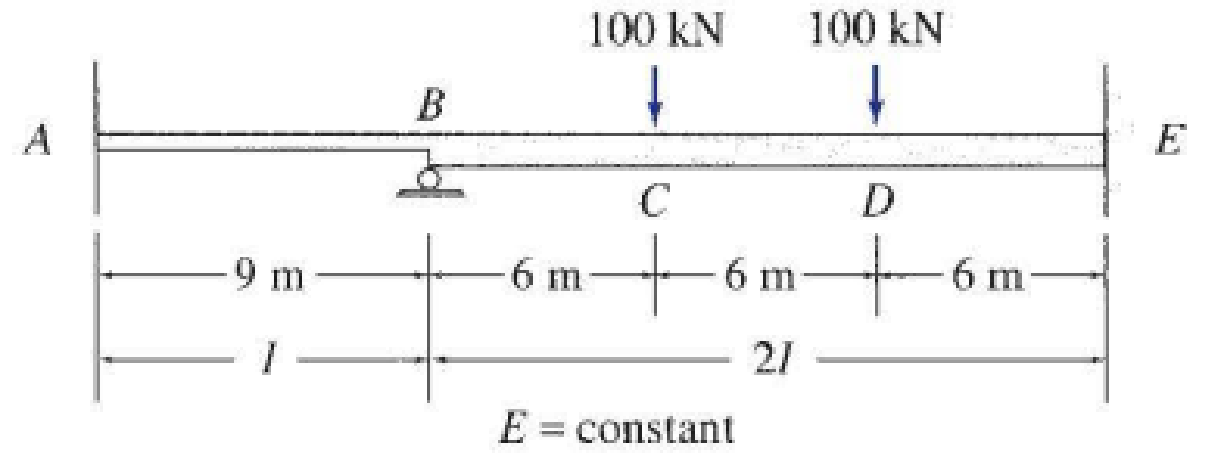# Determine the reactions and draw the shear and bending moment diagrams for the beams shown in Figs. P16.1–P16.5 by using the moment-distribution method. FIG. P16.3

#### Solutions

Chapter
Section
Chapter 16, Problem 3P
Textbook Problem
58 views

## Determine the reactions and draw the shear and bending moment diagrams for the beams shown in Figs. P16.1–P16.5 by using the moment-distribution method.FIG. P16.3

To determine

Find the reaction and plot the shear and bending moment diagram.

### Explanation of Solution

Fixed end moment:

Formula to calculate the relative stiffness for fixed support IL and for roller support (34)(IL).

Formula to calculate the fixed moment for point load with equal length are PL8.

Formula to calculate the fixed moment for point load with unequal length are Pab2L2 and Pa2bL2.

Calculation:

Consider the elastic modulus E of the beam is constant.

Show the free body diagram of the entire beam as in Figure 1.

Refer Figure 1,

Calculate the relative stiffness KBA for member AB of the beam as below:

KBA=I9

Calculate the relative stiffness KBC for member BC of the beam as below:

KBC=(2I18)=I9

In the above beam, only joint B is free to rotate. Hence, calculate the distribution factor at joint C.

Calculate the distribution factor DFCA for member AC of the beam.

DFBA=KBAKBA+KBC

Substitute I9 for KBA and I9 for KBC.

DFBA=I9I9+I9=0.5

Calculate the distribution factor DFBC for member BC of the beam.

DFBC=KBCKBA+KBC

Substitute I9 for KBA and I9 for KBC.

DFBC=I9I9+I9=0.5

Check for sum of distribution factor as below:

DFBA+DFBC=1

Substitute 0.5 for DFAB and 0.5 for DFBC.

0.5+0.5=1

Hence, OK.

Calculate the fixed end moment for AB.

FEMAB=0

Calculate the fixed end moment for BA.

FEMBA=0

Calculate the fixed end moment for BE.

FEMBE=100×6×122182+100×12×62182=400kNm

Calculate the fixed end moment for EB.

FEMEB=100×12×62182100×6×122182=400kNm

Show the calculation of final moments using moment distribution method as in Table 1.

Show the section free body diagram of the member AB and BE as in Figure 2.

Consider the member AB of the beam:

Calculate the vertical reaction at the left end of the joint B by taking moment about point A.

+MA=0By,L(9)+100+200=0By,L=3009By,L=33.33kN

Calculate the horizontal reaction at point A by resolving the horizontal equilibrium.

+Fx=0Ax=0

Calculate the vertical reaction at point A by resolving the vertical equilibrium.

+Fy=0Ay+By,L=0Ay+33

### Still sussing out bartleby?

Check out a sample textbook solution.

See a sample solution

#### The Solution to Your Study Problems

Bartleby provides explanations to thousands of textbook problems written by our experts, many with advanced degrees!

Get Started

Find more solutions based on key concepts
In your own words, explain what we mean by mechanics.

Engineering Fundamentals: An Introduction to Engineering (MindTap Course List)

Create the relational diagram to show the relationship between STORE and REGION.

Database Systems: Design, Implementation, & Management

What is cast iron?

Precision Machining Technology (MindTap Course List)

solid-state media (376) a. storage method in which the magnetic particles are aligned horizontally around a dis...

Enhanced Discovering Computers 2017 (Shelly Cashman Series) (MindTap Course List)

Explain the differences between a Gantt chart and a PERT/CPM chart

Systems Analysis and Design (Shelly Cashman Series) (MindTap Course List)

Convert P = 5.00 atm into Pa, bar, and psia.

Fundamentals of Chemical Engineering Thermodynamics (MindTap Course List)

What problems may result by using an electrode at too low of a current setting?

Welding: Principles and Applications (MindTap Course List)

If your motherboard supports ECC DDR3 memory, can you substitute non-ECC DDR3 memory?

A+ Guide to Hardware (Standalone Book) (MindTap Course List)